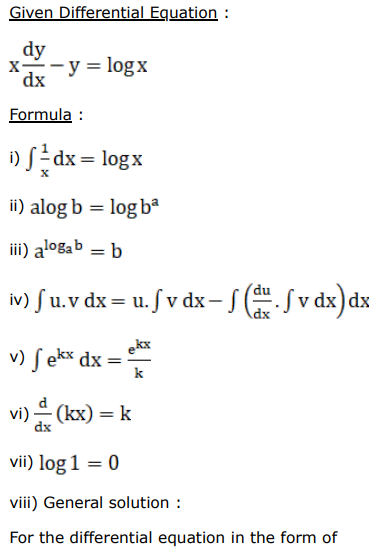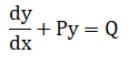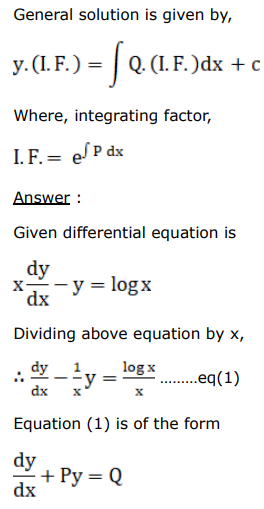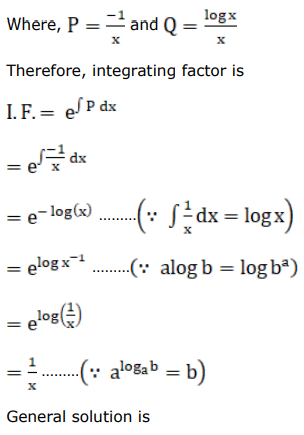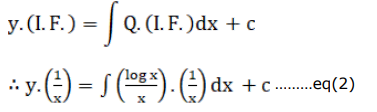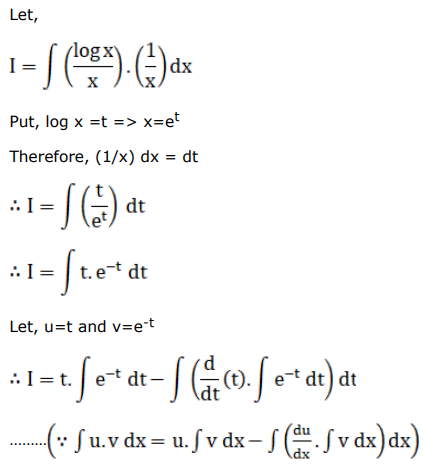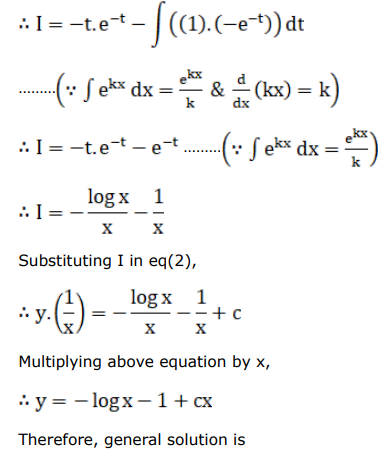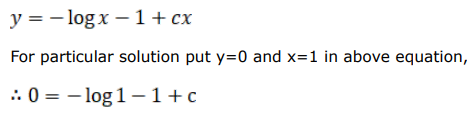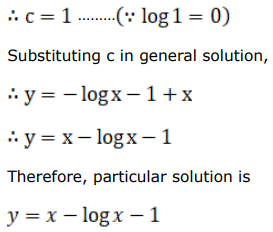# Find a particular solution satisfying the given condition for each of the following differential equations.

Question:

Find a particular solution satisfying the given condition for each of the following differential equations.

$\mathrm{x} \frac{\mathrm{dy}}{\mathrm{dx}}-\mathrm{y}=\log \mathrm{x}$, given that $y=0$ when $x=1$

Solution: